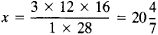# Chain Rule

(redirected from Chain rule (several variables))
Also found in: Dictionary.

## chain rule

[′chān ‚rül]
(mathematics)
A rule for differentiating a composition of functions: (d/dx) ƒ (g (x)) = ƒ′(g (x))· g ′(x).
McGraw-Hill Dictionary of Scientific & Technical Terms, 6E, Copyright © 2003 by The McGraw-Hill Companies, Inc.
The following article is from The Great Soviet Encyclopedia (1979). It might be outdated or ideologically biased.

## Chain Rule

a method given in old arithmetic textbooks for converting the measures of one system into the measures of another system by means of a third system.

Suppose, for example, we want to know the number of vershki equal to 3 feet if 1 foot is equal to 12 inches and 28 inches are equal to 16 vershki. To apply the chain rule, we write the elements of the problem in the following form:

 x vershki 3 feet 1 foot 12 inches 28 inches 16 vershki

The required number of vershki is obtained by dividing the product of the numbers in the right-hand column by the product of the known numbers in the left-hand column:The Great Soviet Encyclopedia, 3rd Edition (1970-1979). © 2010 The Gale Group, Inc. All rights reserved.
Site: Follow: Share:
Open / Close